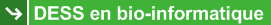Random tree generator

 This program generates k random phylogenetic trees with n leaves (i.e., species, taxa) and an average branch length l using the random tree generation procedure described by Kuhner and Felsenstein (1994). The branch lengths of trees are computed using an exponential distribution. The branch lengths are multiplied by 1+ax, where the variable x is obtained from an exponential distribution (P(x>k) = exp(-k)), and the constant a is a tuning factor for the deviation intensity (as in Guindon and Gascuel (2002), the value of a is fixed to 0.8). The random trees generated by this procedure have depth of O(log (n)).

 Number of trees k = (max = 1000)
 Number of leaves n = (max = 500)
 Average branch length l = (default = 1.0)

 References : Kuhner, M., and J. Felsenstein. 1994. A simulation comparison of phylogeny algorithms under equal and unequal evolutionary rates. Mol. Biol. Evol. 11:459-468. Guindon, S., and O. Gascuel. 2002. Efficient biased estimation of evolutionary distances when substitution rates vary across sites. Mol. Biol. Evol. 19:534-543.

This site has been visited 711027 times since Friday, November 25, 2005.

Boc, A., Diallo, Alpha B. and Makarenkov, V. (2012), T-REX: a web server for inferring, validating and visualizing phylogenetic trees and networks, Nucleic Acids Research, 40(W1), W573-W579.

 Copyright © 2005 Université du Québec à Montréal (UQAM)Webmasters : Alix Boc and Alpha Boubacar Diallo